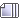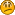Author Topic: Water Shader  (Read 7853 times)AGP

•• Posts: 1620« Reply #15 on: September 19, 2011, 04:52:01 am »
Hey, I seem to have found a nice shader. Problem is, for some reason it causes the world y to be flipped (or perhaps rotated 180 degrees around Z, I'm not sure which but -y is pointing down). The other problem is that nothing I do with the camera now changes the look of the water plane. I've added a billboarded plane to the world. If I move the camera in, the plane grows, if I move it out it shrinks. But the water plane remains unchanged. Very odd. Any insight would be appreciated.

UPDATE: This is the line that holds the water plane in place. But why would anyone write that? What's there to gain with holding it in place?
gl_Position = gl_ProjectionMatrix * gl_Vertex;
UPDATE 2: Apparently you need to write into gl_Position. But what can be passed that doesn't hold the water plane in place?

Code: [Select]
varying vec4 texcoord;
//varying vec4 position; //position is equal to texture coordinate :/ (one image plane)
//vec4 gl_ClipVertex;

void main() {
texcoord = gl_MultiTexCoord0;
// Transforming The Vertex

//position = gl_Vertex;

gl_Position = gl_ProjectionMatrix * gl_Vertex;

//clipping test
//vec3 screen_pos = gl_Position.xyz / gl_Position.w;
//gl_ClipDistance = screen_pos.x + 0.5; //required for clip planes in shader
}

The fragment:
Code: [Select]
//#version 120

uniform float timer;
uniform sampler2D colorMap;
uniform sampler2D reflectedColorMap;
uniform sampler2D stencilMap;
uniform vec3 water_color;
varying vec4 texcoord;

void main () {
//vec3 water_color = vec3(0.0, 0.4, 0.2); //0.22,0.2 (now uniform through config)
float in_water; //'boolean'

vec4 color;
vec4 stencil = texture2D(stencilMap,texcoord.rg);

if (stencil.r > 0.05) in_water = 1.0;
else in_water = 0.0;

//distortion begin
float x_scale = 1.0;
float z_scale = 1.0;

float used_timer = timer;
float time_scale = 2.0; //2.0
float size_scale = 1.6*6.3; //also dependent on radius

if (stencil.r <= 0.15) {
size_scale *= 6.0;
time_scale *= 1.5;
} else {
size_scale *= stencil.r;
}

//timer needs to be 'in period'
if (stencil.r >= 0.5) { //
x_scale = ( 0.995 + (sin(2.0*time_scale*3.14159*used_timer - sin(0.5*size_scale*3.14159*stencil.g) + (size_scale*3.14159*stencil.g))/100.0)); //scales btw 0.995 and 1.005
} //- sin(0.5*size_scale*3.14159*stencil.b)
z_scale = ( 0.995 + (sin(sin(time_scale*3.14159*used_timer)  + 1.5*sin(0.8*size_scale*3.14159*stencil.b))/150.0));
vec2 disturbed = vec2(x_scale*texcoord.x, z_scale*texcoord.y);

vec4 reflection = texture2D(reflectedColorMap,disturbed.rg);
//if (x_scale + z_scale > 2.00099) reflection *= 1.8; //to monitor effects...!

time_scale = 3.0; //2.0
size_scale = 2.4*6.3 * stencil.r;

//timer needs to be 'in period'
if (stencil.r >= 0.5) { //- sin(0.25*size_scale*3.14159*stencil.g)
x_scale = ( 0.995 + (sin(2.0*time_scale*3.14159*used_timer - sin(0.25*size_scale*3.14159*stencil.g) + size_scale*3.14159*stencil.g)/100.0)); //scales btw 0.995 and 1.005
}
z_scale = ( 0.995 + (sin(sin(time_scale*3.14159*used_timer) + 1.5*sin(size_scale*3.14159*stencil.b))/100.0));
vec2 disturbed_2 = vec2(x_scale*texcoord.x, z_scale*texcoord.y);
//distortion end

vec4 reflection_2 = texture2D(reflectedColorMap,disturbed_2.rg);

reflection = (reflection+reflection_2)/2.0;

//'refraction'(for all under-water)
if (in_water > 0.05) {
float look_up_range = 0.008; //0.005 //0.008
//costs performance! (masking to avoid outside water look-ups, alternative another scene clipping)
if (texture2D(stencilMap,vec2(disturbed.r + look_up_range, disturbed.g + look_up_range)).r > 0.001 &&
texture2D(stencilMap,vec2(disturbed.r - look_up_range, disturbed.g - look_up_range)).r > 0.001 &&
texture2D(stencilMap,vec2(disturbed.r, disturbed.g)).r > 0.001) {
color = texture2D(colorMap,disturbed.rg); //drunken effect without stencil if
} else {
color = texture2D(colorMap,texcoord.rg);
}
} else {
color = texture2D(colorMap,texcoord.rg);
}

//combine reflection and scene at water surfaces
float reflection_strength = 0.5 * (stencil.r-0.1); //0.1, 0.14, 0.16, 0.17, 0.5
float disable_refl = stencil.r-0.1;

if (disable_refl <= 0.0) disable_refl = 0.0;

//times inverted color.r for a stronger reflection in darker water parts!
//used to be 8.0, 6.0, 3.5
vec3 reflection_color = vec3(1.0, 1.0, 1.0);
reflection_color =  reflection_strength * disable_refl * reflection.rgb;// * reflection.rgb * in_water * (1.0-(color.r*color.g*color.b));

//more color in darker water in relation to the reflection
//color darkened
float difference = (reflection_color.r+reflection_color.g+reflection_color.b)/3.0 - (color.r + color.g + color.b)/5.5;
if (difference < 0.0) difference = 0.0;
vec3 regular_color = color.rgb * (1.0-in_water*reflection_strength) + (in_water * ((difference) * water_color));

vec4 out_color = vec4(regular_color, 1.0) + vec4(reflection_color, 1.0);

gl_FragColor = out_color;

//debug section
//gl_FragColor = texture2D(colorMap,texcoord.rg);
//gl_FragColor = stencil;
//gl_FragColor = vec4(vec3(stencil.r), 1.0);
}
« Last Edit: September 19, 2011, 08:31:24 am by AGP »MrM

• byte
•• Posts: 36« Reply #16 on: September 19, 2011, 04:01:40 pm »
Can't seem to get it to work, I'm surely missing something, despite the textures.
Anyways, the flipping might be happening because the normal might not be pointing in the right direction, so try an abs() wherever the 'noise' or 'bump' of the water is controlled, so to speak...

Quote
The other problem is that nothing I do with the camera now changes the look of the water plane.
I'm not sure I quite understand... You mean it's like the water isn't moving? Or it's not bumpy/wavy?

And one more thing... I didn't find any information about lights in the shader. Is the water reflec/refrac taking in any lighting from your world? I'm asking because I'm interested in the result, lights aren't always necessary directly in shader code.AGP

•• Posts: 1620« Reply #17 on: September 19, 2011, 06:11:17 pm »
The water plane is always in the same position relative to the camera. The line that causes this is
Code: [Select]
gl_Position = gl_ProjectionMatrix * gl_Vertex;
I need to pass gl_Position something different, that allows it to move relative to the camera.MrM

• byte
•• Posts: 36« Reply #18 on: September 19, 2011, 07:07:03 pm »
Ah, so even when the plane is bilboarded, the Object3D (plane) moves relative with the camera, but the "water texture" on it, does not?If that's the case, I get your problem... And I'm not sure it's gonna be as simple as passing something in the position line...

uniform mat4 modelViewProjectionMatrix;

And in main:
vec3 position = gl_Vertex;
gl_position = modelViewProjectionMatrix * position;

Just like in EgonOlsen's...
(or you might need to make that one into: gl_ModelViewProjectionMatrix)

If not, I've no clue with just copying and pasting like this... I'll need to make it actually work on my side to do some experimenting.AGP

•• Posts: 1620« Reply #19 on: September 19, 2011, 07:17:24 pm »
No, sorry, it's two different planes. A billboarded one, for testing, and the water plane. As I move the camera, the billboarded plane moves intuitively (but the entire world's y is flipped so that this non-shader plane's -y points down). The water never moves at all. If I pass Config.farPlane, the water plane disappears, other than that the camera's position has no effect on it.AGP

•• Posts: 1620« Reply #20 on: September 19, 2011, 09:20:03 pm »
I'm not sure what's different between
Code: [Select]
gl_Position = gl_ProjectionMatrix * gl_Vertex;

and
Code: [Select]
vec3 position = gl_Vertex;
gl_position = modelViewProjectionMatrix * position;//modelViewProjectionMatrix HASN'T BEEN INITIALIZED, SO I GUESS IT'S gl_ProjectionMatrix
It's pretty much the same thing, isn't it?MrM

• byte
•• Posts: 36« Reply #21 on: September 20, 2011, 12:52:38 am »
Yes, that's a a mistake on my part, I must've read something wrong. I really don't know how can I help you, since I don't really understand. The camera isn't supposed to do anything, and something might need to be called at runtime. I didn't manage to get it working, so I can't really try anything out. I think your best bet would be to wait for a response from one of the more experienced members of the forum...EgonOlsen

••• Posts: 11983« Reply #22 on: September 29, 2011, 10:56:28 pm »
Not really related to this particular problem, but i've just uploaded a preview release of 1.24 here: http://jpct.de/download/beta/jpctapi_124pre1.zip

« Last Edit: September 30, 2011, 08:13:24 am by EgonOlsen »AGP

•• Posts: 1620« Reply #23 on: September 30, 2011, 06:39:20 am »
Awesome, thank you. Did you remember that addition to the docs? And while we're on the subject, why not add the "attribute vec4 tangent;" tidbit to them? Thanks again.AGP

•• Posts: 1620« Reply #24 on: September 30, 2011, 06:41:58 am »
By the way, not to be too pushy, but consider updating the included lwjgl with the current one. :- )EgonOlsen

••